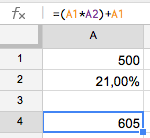# Calculate VAT with Excel

Find out how to calculate VAT using a simple Excel formula. Manage taxes easily form a single spreadsheet.Calculating VAT (Value Added Tax) in Excel is pretty simple as you don’t need to know any specific codes. To calculate VAT you will need to multiply the quantity by the tax percentage converted to a decimal (for example, 21% tax is 0.21, 4% is 0.04…)

`VAT = (price without tax * 0.21)`

This will give us the amount of tax that will need to be added to the initial price to find out the final cost. For example, if a purchase is 500€ with 21% VAT:

`VAT = (500 * 0.21) = 105`

`Price with VAT = 500 + 105 = 605€`

## How to calculate price with VAT in ExcelThis can all be done in a single operation by using the following cell numbers. If cell A1 included the value of the price without VAT (500) and cell A2 the VAT percentage (21%), the formula would be:

`Total price with VAT = (A1*A2) + A1`

## How to calculate the price without VAT in Excel

In the same way, Excel can be used to do the reverse calculation of finding out the inital price by subtracting the VAT percentage. For this, the formula is different as you need to divide instead of multiple and always adding 1 to percentage of VAT:

`Initial price without VAT = price with VAT / (1+0.21)`Following on from the same example we used before, if we put the price with VAT in cell A1 (605) and the VAT percentage in cell A2 (21%), the formula would be:

`Price without VAT = A1 / (1+A2)`

View all the Excel functions.